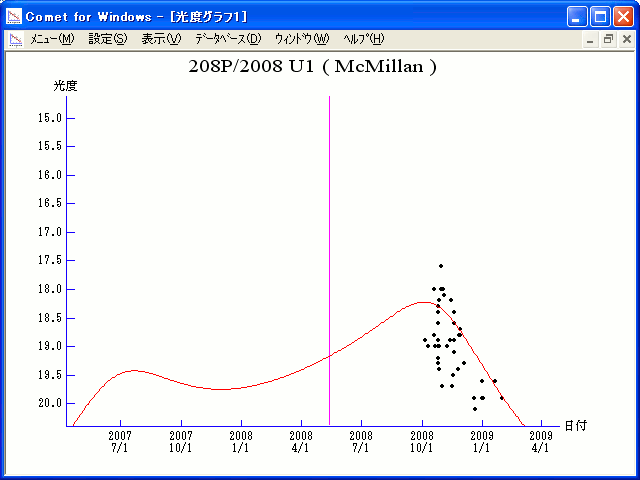# \$B%^%/%_%i%sWB@1(B

208P/McMillan (2008)

 English version Home page Updated on March 8, 2009###\$B%W%m%U%#!<%k(B

 \$BId9f(B 208P/2008 U1 \$BH/8+F|(B 2008\$BG/(B10\$B7n(B19\$BF|(B \$BH/8+8wEY(B 18.9\$BEy(B \$BH/8+ Robert S. McMillan (Kitt Peak)

###\$B50F;MWAG(B

```                    Epoch = 2008 May  14.0 TT
T = 2008 May  13.2573 TT         Peri. = 310.4836
e = 0.374407                     Node  =  36.4178  2000.0
q = 2.524827 AU                  Incl. =   4.4128
a =  4.035895 AU    n = 0.1215610    P =   8.11 years
```

###\$B8wEYJQ2=(B

```        m1 = 12.7 + 5 log\$B&\$(B + 10.0 log r
```##### \$B50F;MWAG\$O!"(BIAUC 9000\$B\$K7G:\\$5\$l\$?\$b\$N\$G\$9!#(B \$B8wEY%0%i%U\$O(BComet for Windows\$B\$G:n@.\$7\$?\$b\$N\$G\$9!#(B